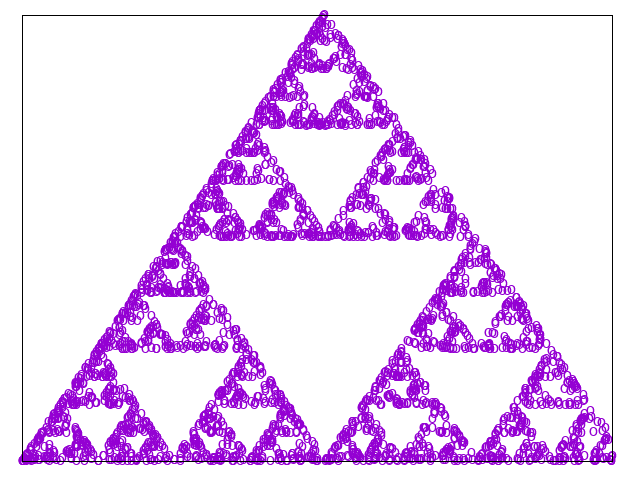Fork me on GitHub
Source file: dex-sierpinski.fut

# Dex: Sierpinski triangle

The following is a port of sierpinski.dx and is fairly straightforward.

``````import "dex-prelude"

type Point = (f64, f64)

def update [n] (points: [n]Point) (key: Key) ((x,y): Point) : Point =
let (x', y') = points[randIdx n key]
in (0.5 * (x + x'), 0.5 * (y + y'))

def runChain 'a (n: i64) (f: Key -> a -> a) (key: Key) (x0: a) : [n]a =
scan' n x0 (many f key)

def trianglePoints : Point =
[(0.0, 0.0), (1.0, 0.0), (0.5, f64.sqrt 0.75)]

def points = unzip (runChain 3000 (update trianglePoints) (newKey 0) (0.0, 0.0))``````

Let’s give it a point plot.

``````> :gnuplot {points=points};
unset xtics
unset ytics
plot points with points pt "o" notitle``````